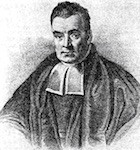# [T15] Bayesian confirmationOne very interesting aspect of statistics is the use of probability in explaining scientific methodology. In particular, the Bayesian approach provides a powerful framework to explain confirmation and many other aspects of scientific reasoning.

On the Bayesian approach, probability is used to measure degrees of belief. Belief does not come in an all-or-nothing manner. If it has been raining heavily the past week, and the clouds have not cleared, you might believe it is going to rain today as well. But you might not be certain that your belief is true, as it is possible that today turns out to be a sunny day. Still, you might decide to bring an umbrealla when you leave home, since you think it is more likely to rain than not. The Bayesian framework is a theory about how we should adjust our degrees of belief in a rational manner. In this theory, the probability of a statement, P(S), indicates the degree of belief an agent has in the truth of the statement S. If you are certain that S is true, then P(S)=1. If you are certain that it is false, then P(S)=0. If you think S is just as likely to be false as it is to be true, then P(S)=0.5.

One important aspect of the theory is that rational degrees of belief should obey the laws of probability theory. For example, one law of probability is that P(S) = 1 - P(not-S). In other words, if you are absolutely certain that S is true, then P(S) should be 1 and P(not-S)=0. It can be shown that if your system of degree of belief deviates from the laws of probability, and you are willing bet according to your beliefs, then you will be willing to enter into bets where you will lose money no matter what.

What is interesting, in the present context, is that

Here, P(H) measures your degree of belief in a hypothesis when you do not know the evidence E, and the conditional probability P(H|E) measures your degree of belief in H when E is known. We might then adopt these definitions :

1. E confirms or supports H when P(H|E) > P(H).
2. E disconfirms H when P(H|E) < P(H).
3. 3. E is neutral with respect to H when P(H|E) = P(H).

As an illustration, consider definition #1. Suppose you are asked whether Mary is married or not. Not knowing her very well, you don't really know. So if H is the statement "Mary is married", then P(H) is around 0.5. Now suppose you observe that she has got kids and has a ring on her finger, and living with a man. This would provide evidence supporting H, even though it does not prove that H is true. The evidence increases your confidence in H, so indeed P(H|E) > P(H). On the other hand, knowing that Mary likes ice-cream probably does not make a difference to your degree of belief in H. So P(H|E) is just the same as P(H), as in definition #3.

One possible measure of the amount of confirmation is the value of P(H|E)-P(H). The higher the value, the bigger the confirmation. The famous Bayes theorem says :

P(H|E) = P(E|H)xP(H)/P(E)

So, using Bayes theorem, the amount of confirmation of hypothesis H by evidence E

= P(H|E) - P(H)
= P(E|H) x P(H)/P(E) - P(H)
= P(H) { P(E|H) / P(E) - 1 }

Notice that all else being equal, the degree of confirmation increases when P(E) decreases. In other words, if the evidence is rather unlikely to happen, this provides a higher amount of confirmation. This accords with the intuition that surprising predictions provide more confirmation than commonplace predictions. So this intuition can actually be justified within the Bayesian framework. Bayesianism is the project of trying to make sense of scientific reasoning and confirmation using the Bayesian framework. This approach holds a lot of promise, but this is not to say that it is uncontroversial.